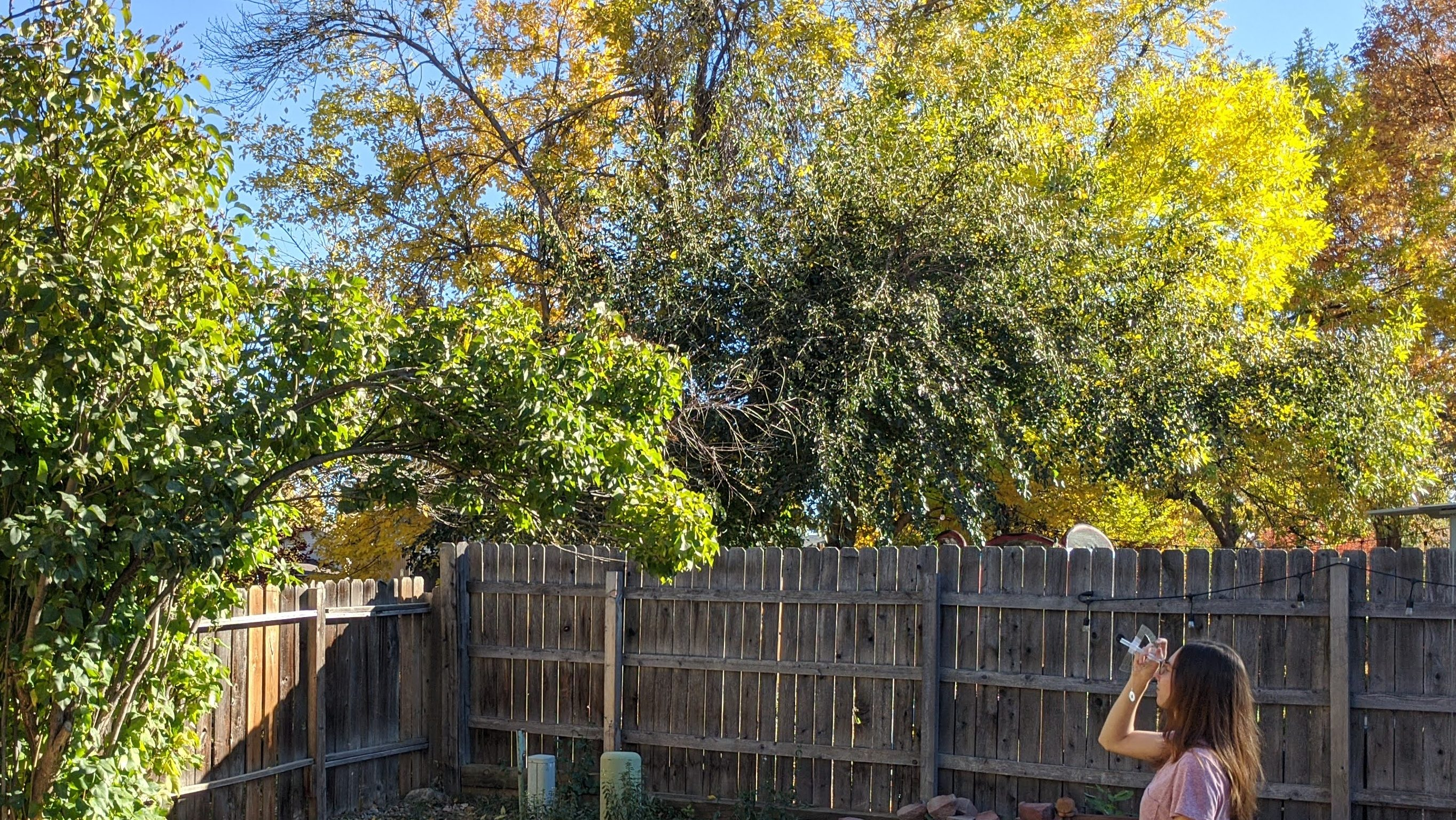Categories

# How to Measure Tree Height: a real-world application of trigonometry and step-by-step guide to make your own inclinometer!

I don’t know about you, but I think math is a lot more interesting when we can apply it to real-world situations! If you’re studying trigonometry, right triangles, or angles, tree height measurements are the perfect applied math activity! In this simple activity you can create your own inclinometer – a device for measuring angle of inclination, and use it to calculate the height of a tree!

## How do you measure tree height?

Unless you are measuring a short seedling, you probably won’t be able to measure tree height directly because you can’t reach! It can be challenging to figure measure something so tall.

An inclinometer is a device fore measuring an angle of inclination above horizontal. We can use inclinometers to help calculate the height of tall objects, such as trees! Using the angle from the inclinometer and the distance from the tree where the measurement was taken, you can calculate the tree height with trigonometry – a branch of mathematics dealing with angles and the sides of triangles.

## How to make your own inclinometer

You can make your very own inclinometer from common household items to use during the tree height activities in this unit!

Materials

• Tape
• Straw
• A small weight (e.g., a button or a washer)
• Scissors

Steps

1. Tape the straw across the center of the protractor at 90̊.
2. Use the scissors to shorten the straw (as needed).
3. Cut a short piece of thread or dental floss.
4. Tie the small weight to the end of the floss.
5. Tie the other end of the floss to the straw.
6. Check that the thread hangs over the 0̊ mark when holding the inclinometer with the straw parallel to the ground. Adjust the position of the thread on the straw as needed.

## How to use a homemade inclinometer

Use your homemade inclinometer to measure the angles to the tops of trees. The homemade inclinometer requires two people to operate – one person to hold the inclinometer while looking at the top of the tree, and one person to read the angle off of the side.

Materials

• Tape measure
• A friend

Directions

1. Stand a short distance away from the tree, far enough away that you can see the top of the tree. This works best if you are on flat ground – not uphill or downhill from the tree. Measure your distance from the tree.
2. Look through the straw with the curved edge of the protractor facing your eyeball. Do not touch the straw to your eyeball.
3. As you look through the straw, tip the inclinometer upwards until you can see the top of the tree through the straw.
4. Hold still and have a friend look at the side of the inclinometer. Have them read the angle where the thread crosses.
5. Measure the height to your eye level.As you look through the straw, tip the inclinometer upwards until you can see the top of the tree through the straw. Have a friend read the angle on the side of the inclinometer where the thread crosses.

## How to calculate tree height

You can use trigonometry to calculate the tree height from the measurements you took with the tape measure and inclinometer. The imaginary horizontal line at eye level, the imaginary line from your eyes to the top of the tree, and the tree itself create a right triangle (see figure below). Right triangles have known relationships between their angles and the lengths of their sides! If you know two angles and the length of a side of the triangle, you can calculate the length of the other sides of the triangle. Look at the following diagram then check out the example calculation below!

#### Example Calculation

Question: I want to know the height of a tree in my back yard. When I was standing 15 feet away from the tree, I used my inclinometer to find an angle to the top of the tree of 20̊. The height to my eye level is 5.2 feet.

distance x TAN(angle) = tree height above eye level

15 feet * TAN (20̊) = 5.5 feet

tree height above eye level + eye level = tree height

5.5 feet + 5.2 feet = 10.7 feet

Find additional readings, activities, and reference websites related to this activity here!

Are you interested in more hands-on science activities? Subscribe or follow Wild Earth Lab using the links below!

#### Subscribe to receive informative blog posts about science, sustainability, and nature delivered straight to your inbox!

Join 150 other subscribers

#### Subscribe to receive informative blog posts about science, sustainability, and nature delivered straight to your inbox!

Join 150 other subscribers